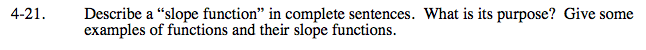### Home > CALC > Chapter 4 > Lesson 4.1.2 > Problem4-21

4-21.Discuss the formal definition of a derivative (slope function) as a limit.

Compare and contrast IROC and AROC (or slope of tangent vs. slope of secant) and how that relates to slope functions.

Discuss some practical uses of slope functions; such as, finding where the original function is increasing, decreasing or at a local maximum or local minimum.

Discuss how slope functions appear in motion problems; What does slope on a distance graph represent? What does slope on a velocity graph represent?